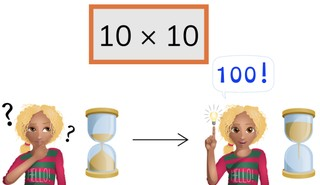Automaticity 10 times table

# Automaticity 10 times table

Students learn to memorize the 10 times table.8,000 schools use Gynzy92,000 teachers use Gynzy1,600,000 students use Gynzy

## General

The students memorize the 10 times table.

## Standards

CCSS.Math.Content.3.OA.C.7

## Learning objective

Students will be able to memorize the 10 times table and quickly solve its products.

## Introduction

Discuss with the students which answers are in the 10 times table and drag the answers to the right column.

## Instruction

Have the students first solve the products from the 10 times table within 5, 4, and 3 seconds. Then show the pile of cards. Explain that you are going to start the timer and drag the cover away to show the first problem. Have the students give the right answer to the problem and then drag the card aside to show the next problem. Try to complete this within the time given by the timer.

Check whether the students have memorized the 10 times table with the following question:
- Why is it important to memorize the 10 times table?

## Quiz

The students practice the 10 times table with 3 exercises.

## Closing

Discuss once again the importance of memorizing the 10 times table. To close, have all of the students stand up and spin the wheel. When the answer to the problem is larger than 50 they jump to the right, if it is smaller they jump to the left.

## Teaching tips

Give students that have difficulty memorizing the 10 times table a printed 10 times table to practice with. Have them practice in pairs by practicing products of the 10 times table in shorter and shorter amounts of time.

## Instruction materials

You may print out a copy of the 10 times table.

### The online teaching platform for interactive whiteboards and displays in schools

• Save time building lessons

• Manage the classroom more efficiently

• Increase student engagement